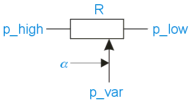﻿ 20-sim webhelp > Library > Iconic Diagrams > Electric > Components > potentiometer

# potentiometer

## Library

Iconic Diagrams\Electric\Components

## Use

Domains: Continuous. Size: 1-D. Kind: Iconic Diagrams (Electric).

## Description

This model represents an ideal potentiometer.It can have various forms of causality. The equations are:

R1 = (1 - alpha) * R;

R2 = alpha * R;

p_high.u - p_var.u = p_high.i * R1;

p_var.u - p_low.u = p_low.i * R2;

p_high.i + p_var.i = p_low.i;

where alpha denotes the position of the indicator and can vary from zero to one.

## Interface

 Ports Description p_high, p_low p_var Both terminals of the resistor. Indicator terminal. Causality indifferent Parameters R alpha Resistance [Ohm]. Indicator position (0 <= alpha <= 1).